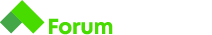# Above the Main Diagonal Java need help

Здравейте,
Моля за съдействие с тази задача. Опитвам някакви решения, но не стигам до никъде. Ето го условието.

# Description

You are given a number `N` . Using it, create a square matrix of numbers, formed by powers of 2 and find the sum of the numbers above the main diagonal, excluding the diagonal itself.

# Input

• On the first line, read the number `N` - the number of rows and columns.

# Output

Print to the standard output.

• On a single line print the sum of the numbers above the main diagonal excluding the diagonal.

# Constraints

• `N` can get as big as `30` .

## Input

``````4
``````

## Output

``````70
``````

## Explanation

With `N` equal to 4, we will have a matrix that looks like this:

`````` 1  2  4  8
2  4  8 16
4  8 16 32
8 16 32 64
``````

Благодаря предварително.

Аз я реших с вложен цикъл като симулирам реда от матрицата но не го запаметявам,ами с всеки оборот на цикъла реда се сменя до n ред и стария се изтрива.
На всяка врътка сумирам реда,като намалявам елементите с -1 за всеки цикъл на цикъла.
Предпоследния ред просто изваждам от масива последния елемент и го добавям към общата сума.

За съжаление решението ми е на Python не на Java.

``````n = int(input())
l =*n
s = 0
custom_index = 0
for i in range(1,n):
number = l
if i == 1:
custom_index = 1
else:
custom_index = 0
for j in range(custom_index,n):
l[j] = number * 2
number *= 2
if i == n - 1:
s += l[-1]
else:
s += sum(l[i:])
print(s)
``````
1 Like
``````import java.io.BufferedReader;
import java.io.IOException;

public class AboveTheMainDiagonal {

public static void main(String[] args) throws IOException {
long value=0;
for (int i = 1; i < n; i++)
for (int j = i; j < n; j++)
value+= (long)Math.pow(2,i+j-1);
System.out.println(+value);

}
}
``````

Ето едно чуждо решение на Джава,което можеш да си разгледаш с дебъгера.

2 Likes

Страхотно. Ще разгледам подробно, тъкмо има функции, които не съм използвала.
Много благодаряТова което виждам аз е подобно на моето решение,реално матрицата никога не се създава,както е на примерната задача ами ред по ред се симулира и сумира с n - 1 елемента.

Помисли над тази логика и ако не можеш да решиш една задача,просто я остави за другия ден.

1 Like

Разгледах я и я съкратих малко. Търсех някакво по-сложно решение явно, но сега като го дебъгнах го разбрах. Ето и моят вариант:
import java.util.Scanner;
public class AbovetheMainDiagonal {
public static void main(String[] args){
Scanner scanner = new Scanner(System.in);
int n = Integer.parseInt(scanner.nextLine());
long value=0;
for (int i = 1; i < n; i++)
for (int j = i; j < n; j++)
value+= (long)Math.pow(2,i+j-1);
System.out.println(value);
}
}

2 Likes

Малеее, аз правя всичко с int и се чудя защо стигам само до половината. Без да си искате ми помогнахте доста.

1 Like

@kirilova.gergana55 , благодаря, твоето решение ми помогна за Python варианта``````n = int(input())
result_value = 0
for x in range(1, n):
for y in range(x, n):
result_value += pow(2, x+y-1)
print(result_value)
``````
1 Like